# Fraction calculator

The calculator performs basic and advanced operations with fractions, expressions with fractions combined with integers, decimals, and mixed numbers. It also shows detailed step-by-step information about the fraction calculation procedure. Solve problems with two, three, or more fractions and numbers in one expression.

## Result:

### 1/6 : 1/2 = 1/3 ≅ 0.3333333

Spelled result in words is one third.

### How do you solve fractions step by step?

1. Divide: 1/6 : 1/2 = 1/6 · 2/1 = 1 · 2/6 · 1 = 2/6 = 2 · 1 /2 · 3 = 1/3
Dividing two fractions is the same as multiplying the first fraction by the reciprocal value of the second fraction. The first sub-step is to find the reciprocal (reverse the numerator and denominator, reciprocal of 1/2 is 2/1) of the second fraction. Next, multiply the two numerators. Then, multiply the two denominators. In the following intermediate step, cancel by a common factor of 2 gives 1/3.
In other words - one sixth divided by one half = one third.

#### Rules for expressions with fractions:

Fractions - simply use a forward slash between the numerator and denominator, i.e., for five-hundredths, enter 5/100. If you are using mixed numbers, be sure to leave a single space between the whole and fraction part.
The slash separates the numerator (number above a fraction line) and denominator (number below).

Mixed numerals (mixed fractions or mixed numbers) write as integer separated by one space and fraction i.e., 1 2/3 (having the same sign). An example of a negative mixed fraction: -5 1/2.
Because slash is both signs for fraction line and division, we recommended use colon (:) as the operator of division fractions i.e., 1/2 : 3.

Decimals (decimal numbers) enter with a decimal point . and they are automatically converted to fractions - i.e. 1.45.

The colon : and slash / is the symbol of division. Can be used to divide mixed numbers 1 2/3 : 4 3/8 or can be used for write complex fractions i.e. 1/2 : 1/3.
An asterisk * or × is the symbol for multiplication.
Plus + is addition, minus sign - is subtraction and ()[] is mathematical parentheses.
The exponentiation/power symbol is ^ - for example: (7/8-4/5)^2 = (7/8-4/5)2

#### Examples:

subtracting fractions: 2/3 - 1/2
multiplying fractions: 7/8 * 3/9
dividing Fractions: 1/2 : 3/4
exponentiation of fraction: 3/5^3
fractional exponents: 16 ^ 1/2
adding fractions and mixed numbers: 8/5 + 6 2/7
dividing integer and fraction: 5 ÷ 1/2
complex fractions: 5/8 : 2 2/3
decimal to fraction: 0.625
Fraction to Decimal: 1/4
Fraction to Percent: 1/8 %
comparing fractions: 1/4 2/3
multiplying a fraction by a whole number: 6 * 3/4
square root of a fraction: sqrt(1/16)
reducing or simplifying the fraction (simplification) - dividing the numerator and denominator of a fraction by the same non-zero number - equivalent fraction: 4/22
expression with brackets: 1/3 * (1/2 - 3 3/8)
compound fraction: 3/4 of 5/7
fractions multiple: 2/3 of 3/5
divide to find the quotient: 3/5 ÷ 2/3

The calculator follows well-known rules for order of operations. The most common mnemonics for remembering this order of operations are:
PEMDAS - Parentheses, Exponents, Multiplication, Division, Addition, Subtraction.
BEDMAS - Brackets, Exponents, Division, Multiplication, Addition, Subtraction
BODMAS - Brackets, Of or Order, Division, Multiplication, Addition, Subtraction.
GEMDAS - Grouping Symbols - brackets (){}, Exponents, Multiplication, Division, Addition, Subtraction.
Be careful, always do multiplication and division before addition and subtraction. Some operators (+ and -) and (* and /) has the same priority and then must evaluate from left to right.

## Fractions in word problems:

• Golf ballsOf the 28 golf balls, 1/7 are yellow. How many golf balls are yellow? Use the model to help you. Enter your answer in the box.
• Blue silk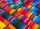There are 4 rolls of blue silk. Each roll holds 6 3/4 yd of silk. It takes 3/5 of a yard to make one necktie. How many necktie can be made from the 4 rolls of blue silk?
• The rope 2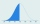The rope in the school gym is 10 ½ feet long. To make it easier to climb, Coach Hill tied a knot in the rope every ¾ foot. How many knots are in the rope?
• Chester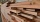Chester has a piece of wood measuring 1 2/3 cm. He needs to cut it to pieces measuring 3/4 cm long. How many pieces of wood did Chester cut?
• Kate shares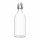Kate shares a 64-ounce bottle of apple cider with five friends. Each person's serving will be the same number of ounces. Between what two whole number of ounces will each person's serving to be? Explain using division.
• Brooke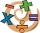Brooke divided 2/3 by 1/6 and got 1/9. Her classmate told her that she isn’t correct. What do you think went wrong with Brooke’s solution? Explain your answer in 2 - 3 sentences.
• Licorice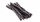A piece of licorice is 2/3 yard long.  If it is cut into 10 equal pieces, how long will each piece of licorice be?
• Larry 2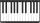Larry spends half of his workday teaching piano lessons. If he sees 6 students, each for the same amount of time, what fraction of his workday is spent with each student?
• Halves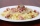How many halves are there in  5 3/4?
• Self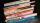A math textbook is 2 2/9 inches thick. How many of these books will fit on a 120-inch self?
• James 2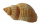James collected 24 seashells on the seashore to be shared with his 2 friends. What fractional part of the seashells will each one get if it is distributed evenly? ​
• Rewrite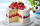Rewrite the following as division statement  if there are 8 cakes and each person get 1/12 of a cake how many people get cake?
• Scale of the mapDetermine the map's scale, which is the actual distance of 120 km l represented by a segment long 6 cm.